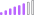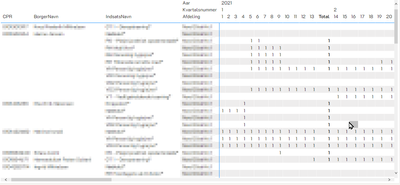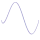cancel
Showing results for
Did you mean:

Fabric is Generally Available. Browse Fabric Presentations. Work towards your Fabric certification with the Cloud Skills Challenge.Post Patron

## Measure is working PERFECT - but doesn't give the total?

My data looks something like this:

 ID Date Service Department Pause 1 01012022 This That False 1 02012022 This That True 2 01012022 Other Somewhere True 2 02012022 Other Somewhere True 3 01012022 Dis There False 3 02022022 Dis There False

I've made a matrix visual that has the first four columns as columns, week number as row, and for the value, I did this:

``````VAR __Active = CALCULATE(COUNTROWS(DISTINCT(TABLE[Date])))
var __Pause = CALCULATE(COUNTROWS(DISTINCT(TABLE[Pause])), TABLE[Pause] = FALSE())

RETURN
IF(__Pause = 1 && __Active > 0, 1)``````

1. There are only two dates I'm looking at - the table is filtered on monday and tuesday only

2. __Active will show me if at least one of the dates exists for a given week number

3. __Pause shows me if at least one of the pause values is FALSE()

4. if __Pause and __Active, then 1 - there's no need for else here

Now, this works perfectly - you can see my matrix visual here:the 1 is to show me if the criteria is met during a given week, per person, service, and department. I've validated the data, and it's showing me exactly what I need to see.

But I really need to add up all those 1's, but I'm getting no totals at all - and if I try grouping things, I never get more than 1. Any idea of what I can do here?

1 ACCEPTED SOLUTIONCommunity Support

Hi @grggmrtn ,

This is a normal problem when user calculate some expressions via measure. The reason is the total column has not the same filter context as the sub items but calculate by same DAX.

So please consider using HASONEVALUE() to let the measure know when use expression for sub items and when use expression for total.

``````Measure =
VAR _Active =
CALCULATE( COUNTROWS( DISTINCT( TABLE[Date] ) ) )
VAR _Pause =
CALCULATE( COUNTROWS( DISTINCT( TABLE[Pause] ) ), TABLE[Pause] = FALSE() )
VAR _summarize =
SUMMARIZE(
table,
[CPR],
[BorgerNavn],
[IndsatsNavn],
"Active", _Active,
"Pause", _Pause
)
ADDCOLUMNS( _summarize, "if", IF( [Pause] = 1 && [Active] > 0, 1 ) )
VAR _sum =
RETURN
IF(
HASONEVALUE( 'table'[Afdeling] ),
IF( __Pause = 1 && __Active > 0, 1 ),
_sum
)
``````

Best Regards

Community Support Team _ chenwu zhu

If this post helps, then please consider Accept it as the solution to help the other members find it more quickly.

2 REPLIES 2Community Support

Hi @grggmrtn ,

This is a normal problem when user calculate some expressions via measure. The reason is the total column has not the same filter context as the sub items but calculate by same DAX.

So please consider using HASONEVALUE() to let the measure know when use expression for sub items and when use expression for total.

``````Measure =
VAR _Active =
CALCULATE( COUNTROWS( DISTINCT( TABLE[Date] ) ) )
VAR _Pause =
CALCULATE( COUNTROWS( DISTINCT( TABLE[Pause] ) ), TABLE[Pause] = FALSE() )
VAR _summarize =
SUMMARIZE(
table,
[CPR],
[BorgerNavn],
[IndsatsNavn],
"Active", _Active,
"Pause", _Pause
)
ADDCOLUMNS( _summarize, "if", IF( [Pause] = 1 && [Active] > 0, 1 ) )
VAR _sum =
RETURN
IF(
HASONEVALUE( 'table'[Afdeling] ),
IF( __Pause = 1 && __Active > 0, 1 ),
_sum
)
``````

Best Regards

Community Support Team _ chenwu zhu

If this post helps, then please consider Accept it as the solution to help the other members find it more quickly.Super User

@grggmrtn This looks like a measure totals problem. Very common. See my post about it here: https://community.powerbi.com/t5/DAX-Commands-and-Tips/Dealing-with-Measure-Totals/td-p/63376

Also, this Quick Measure, Measure Totals, The Final Word should get you what you need:
https://community.powerbi.com/t5/Quick-Measures-Gallery/Measure-Totals-The-Final-Word/m-p/547907

Become an expert!: Enterprise DNA
External Tools: MSHGQM
Latest book!:
Mastering Power BI 2nd EditionDAX is easy, CALCULATE makes DAX hard...Announcements#### Power BI Monthly Update - November 2023

Check out the November 2023 Power BI update to learn about new features.#### Fabric Community News unified experience

Read the latest Fabric Community announcements, including updates on Power BI, Synapse, Data Factory and Data Activator.#### Exclusive opportunity for Women!

Join us for a free, hands-on Microsoft workshop led by women trainers for women where you will learn how to build a Dashboard in a Day!#### The largest Power BI and Fabric virtual conference

130+ sessions, 130+ speakers, Product managers, MVPs, and experts. All about Power BI and Fabric. Attend online or watch the recordings.Top Solution Authors
Top Kudoed Authors
Users online (2,573)